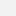# Humboldt-Universität zu Berlin - Faculty of Mathematics and Natural Sciences - Strukturforschung / Elektronenmikroskopie

Matlab programs

## Matlab programs

The following list contains a few simple Matlab-functions, which have been written to illustrate the subjects of the lectures. Some of these functions contain some very simple graphical user interface (GUI) features that allow online modification of parameters without having to change a single line of Matlab code.
In order to keep the code readable by novice Matlab users, the implementation of these GUI-elements has been done in the most simple way, without using any of the GUI design tools built into Matlab. If not instructed otherwise these functions should be called without any input parameters.test_FFT.m: This function demonstrates the relation between real- and reciprocal space. The user may adjust width and position of a Gaussian or top hat aperture function and observe corresponding changes in amplitude and phase of the resulting FFT.Note that changing the width of the function in real space changes its width in reciprocal space acording to the uncertainty principle, keeping the product of the widths of both functions the constant. Because originally written for electron micorscopy, the nomenclature for k used in this k=1/lambda.

 test_FFTbandwidth.m: This function demonstrates the effect that the number of Fourier coefficients has on the resolution of different 1-dimensional functions. While only 2 components are necessary to define the sine wave (offset and wavelength), many more are needed to define the sharp corners of a top hat aperture function. Because originally written for electron micorscopy, the nomenclature for k used in this k=1/lambda.

 test_XtalFFT.m: This function demonstrates the effect that the size of a 1-dimensional crystal (or its shape, when translated to 3 dimensions) has on the Fourier transform of its potential. the user may change the lattice parameter as well as the number of unit cells in the crystal. The sampling applied within this function causes the FFT to converge to that of a perfect crystal, once the number of unit cells reaches 16.Note that the FFT has been normalized, so that its k=0 component does not change with the number of unit cells. Also, because originally written for electron micorscopy, the nomenclature for k used in this k=1/lambda.defocusImage.zip: This function demonstrates the effect which defocus has on images produces with monochromatic light or electrons. It also shows the Fourier transform of various shapes.

 wavelength: computes the wavelength in Angstroem for a given accelerating voltage (in keV). Syntax: lambda = wavelength(E); fwhm: determines the full width at half maximum (FWHM) of a function in which only a single peak is at least twice as high as the rest of the function. The result is given in units of the position vector. Syntax: FWHM = fwhm(x,f); FWHM = fwhm(f); (if the position vector is omitted, f is expected to be sampled equidistantly with sampling 1) smoothEdges: smoothes the edges of all the images in a stack of image (i.e. a 3-dimensional data cube). A single image may be passed into the function as well, of course. The purpose of this smoothing is the reduction of artefacts when computing FFTs. The second parameter (Nedge) determines, how many pixels into the image the edges will be smoothed. Syntax: newImg  =  smoothEdges(img,Nedge); newImgStack  =  smoothEdges(imgStack,Nedge);